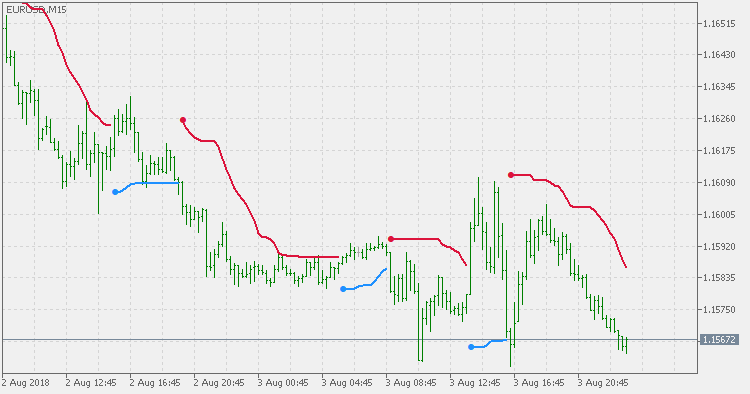Interesting script?
So post a link to it -
let others appraise it

You liked the script? Try it in the MetaTrader 5 terminal# STD Trend Envelopes of Averages - indicator for MetaTrader 5

Views:
1264
Rating:
Published:
2018.08.07 12:57

Trend Envelopes indicator is calculated based on a % of price change. That % is then used to determine if there is a new trend or trend remains the same.

This version is using Standard Deviation for the price change calculation instead and is adding prices smoothing prior to be used in calculations. The smoothing can be done using one of the 4 averages types:

• Simple Moving Average
• Exponential Moving Average
• Smoothed Moving Average
• Linear Weighted Moving AverageSTD Trend Envelopes - RSI

This version of Trend Envelopes RSI indicator differs in one significant point: instead of using fixed value (5) for envelopes calculation, standard deviation (adjusted by multiplier) of the calculated RSI is used for envelopes calculation.STD Trend Envelopes

Trend Envelopes indicator that uses Standard Deviation for the price change calculation.ATR Trend Envelopes of Averages

Trend Envelopes indicator that uses ATR for the price change calculation and is adding prices smoothing prior to be used in calculations.iTrend JMA

Very well known iTrend indicator but that uses JMA (Jurik Moving Average) for calculation.